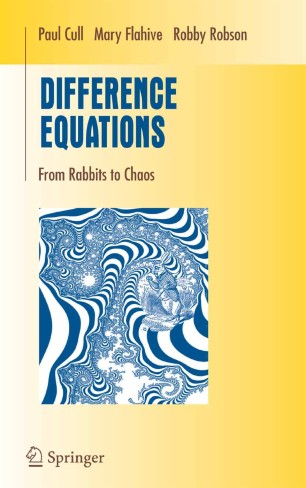# Difference Equations

## From Rabbits to ChaosTextbook

Part of the Undergraduate Texts in Mathematics book series (UTM)

1. Front Matter
Pages i-xiii
2. Pages 1-10
3. Pages 11-31
4. Pages 33-65
5. Pages 67-99
6. Pages 101-135
7. Pages 137-178
8. Pages 179-216
9. Pages 217-251
10. Pages 253-295
11. Pages 297-336
12. Back Matter
Pages 337-392

### Introduction

Difference equations are models of the world around us. From clocks to computers to chromosomes, processing discrete objects in discrete steps is a common theme. Difference equations arise naturally from such discrete descriptions and allow us to pose and answer such questions as: How much? How many? How long? Difference equations are a necessary part of the mathematical repertoire of all modern scientists and engineers.

In this new text, designed for sophomores studying mathematics and computer science, the authors cover the basics of difference equations and some of their applications in computing and in population biology. Each chapter leads to techniques that can be applied by hand to small examples or programmed for larger problems. Along the way, the reader will use linear algebra and graph theory, develop formal power series, solve combinatorial problems, visit Perron—Frobenius theory, discuss pseudorandom number generation and integer factorization, and apply the Fast Fourier Transform to multiply polynomials quickly.

The book contains many worked examples and over 250 exercises. While these exercises are accessible to students and have been class-tested, they also suggest further problems and possible research topics.

Paul Cull is a professor of Computer Science at Oregon State University. Mary Flahive is a professor of Mathematics at Oregon State University. Robby Robson is president of Eduworks, an e-learning consulting firm. None has a rabbit.

### Keywords

Finite Matrix algebra difference equation equation function graph theory linear algebra mathematics

#### Authors and affiliations

1. 1.Dept. Computer Science Dearborn HallOregon State UniversityCorvallisUSA
2. 2.Dept. Mathematics Kidder HallOregon State UniversityCorvallisUSA
3. 3.EduworksCorvallisUSA

### Bibliographic information

• Book Title Difference Equations
• Book Subtitle From Rabbits to Chaos
• Authors Paul Cull
Mary Flahive
Robby Robson
• Series Title Undergraduate Texts in Mathematics
• DOI https://doi.org/10.1007/0-387-27645-9
• Publisher Name Springer, New York, NY
• eBook Packages Mathematics and Statistics Mathematics and Statistics (R0)
• Hardcover ISBN 978-0-387-23233-1
• Softcover ISBN 978-0-387-23234-8
• eBook ISBN 978-0-387-27645-8
• Series ISSN 0172-6056
• Edition Number 1
• Number of Pages XIII, 392
• Number of Illustrations 0 b/w illustrations, 0 illustrations in colour
• Topics
• Buy this book on publisher's site

## Reviews

From the reviews:

"The book under review is written for advanced undergraduate majors. … This book is well written and easy to read, with a large number of exercises … . Difference equations: from rabbits to chaos is a good text for those who want to teach an introductory course in recurrences from a collection of various classical points of view. … anyone interested in difference equations … will want Cull, Flahive, and Robson as a reference because of its interesting and unique collection of topics." (Shandelle M. Henson, Mathematical Reviews, Issue 2006 j)

"This book is a nice addition to an already nice group of books on difference equations … . would be especially appealing to students who like to compute. … This book contains many interesting examples … . There are over 250 exercises. This book could be used for an upper level undergraduate course or for a beginning graduate level course. … it would be nice to have in one’s personal library." (Allan Peterson, SIAM Review, Vol. 48 (1), 2006)

"Recognizing the increasing importance of difference equation models … the authors aimed to write a book that provides a solid foundation in the field and is accessible to undergraduates. … this text is written in an informal style and with an ‘algorithmic spirit’. … There are many worked examples and the exercise sets are good … . it provides an accessible introduction to the material and a firm foundation for applications in various scientific fields." (Henry Ricardo, MathDL, September, 2005)

"As the authors point out in their introduction this is a textbook for undergraduate students taking introductory courses on discrete mathematics. The text is quite elementary, written for an audience with a preference for computational aspects of mathematics and modelling real world phenomena. This is also reflected by the exercises at the end of each chapter. Starting with simple examples … the reader is lead to basic notions and theorems of basic linear algebra and analysis. Proofs are worked out accurately … ." (Stefan Hilger, Zentralblatt MATH, Vol. 1085, 2006)

"This book is specifically designed for American undergraduate students studying mathematics or computer science; it is part of Springer’s undergraduate texts in mathematics. … It will be useful to have on the bookshelf, and when there is a specific difference equation in front of me it will be my first port of call." (Tony Crilly, The Mathematical Gazette, Vol. 91 (521), 2007)